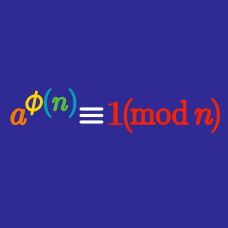Number Theory

# Euler's Theorem Warmup

Is there a positive integer $n$ such that $2^n \equiv 1 \pmod{7} \, ?$

$\frac{1}{15}, \frac{2}{15}, \frac{3}{15}, \ldots, \frac{14}{15}, \frac{15}{15}$ How many of these fractions cannot be reduced?

Is 999999 divisible by 7?

Hint: $999999 = 10^6 - 1.$

What is the last digit of $3^{100} \, ?$

Which of these is congruent to $10^{100} \pmod{11} \, ?$

×